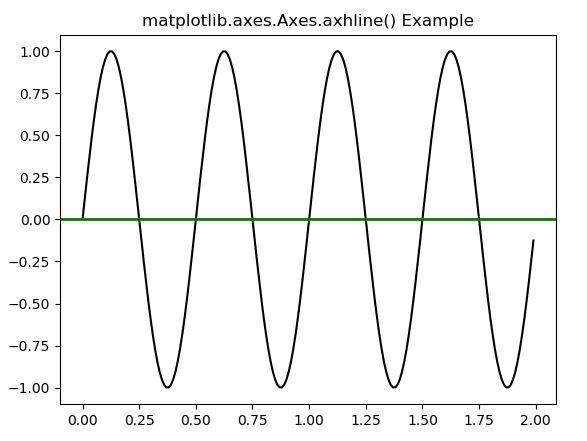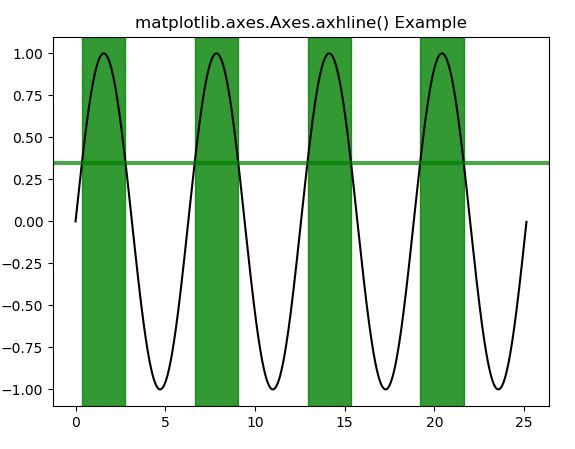Matplotlib.axes.Axes.axhline() in Python

• Last Updated : 13 Apr, 2020

Matplotlib is a library in Python and it is numerical – mathematical extension for NumPy library. The Axes Class contains most of the figure elements: Axis, Tick, Line2D, Text, Polygon, etc., and sets the coordinate system. And the instances of Axes supports callbacks through a callbacks attribute.

matplotlib.axes.Axes.axhline() Function

The Axes.axhline() function in axes module of matplotlib library is used to add a horizontal line across the axis.

Syntax:

Axes.axhline(self, y=0, xmin=0, xmax=1, **kwargs)

Parameters: This method accept the following parameters that are described below:

• y: This parameter is the y position in data coordinates of the horizontal line with default value of 0.
• xmin: This parameter should be between 0 and 1, 0 being the far left of the plot, 1 the far right of the plot.Its default value of 0.
• xmax: This parameter should be between 0 and 1, 0 being the far left of the plot, 1 the far right of the plot. Its default value of 1.

Returns: This returns the following:

• lines:This returns the list of Line2D objects representing the plotted data.

Below examples illustrate the matplotlib.axes.Axes.axhline() function in matplotlib.axes:

Example-1:

 # Implementation of matplotlib functionimport matplotlib.pyplot as pltimport numpy as npimport matplotlib.collections as collections  t = np.arange(0.0, 2, 0.01)s1 = np.sin(4 * np.pi * t)s2 = 0.75 * np.sin(8 * np.pi * t)  fig, ax = plt.subplots()  ax.plot(t, s1, color ='black')ax.axhline(0, color ='green', lw = 2)ax.set_title('matplotlib.axes.Axes.axhline() Example')plt.show()

Output:Example-2:

 # Implementation of matplotlib functionimport matplotlib.pyplot as pltimport matplotlib.tri as mtriimport numpy as np  fig, ax = plt.subplots()x = np.arange(0, 8 * np.pi, 0.01)y = np.sin(x)ax.plot(x, y, color ='black')  threshold = 0.35ax.axhline(threshold, color ='green',           lw = 3, alpha = 0.7)  ax.fill_between(x, 0, 1, where = y > threshold,                color ='green', alpha = 0.8,                 transform = ax.get_xaxis_transform())  ax.set_title('matplotlib.axes.Axes.axhline() Example')plt.show()

Output:Attention geek! Strengthen your foundations with the Python Programming Foundation Course and learn the basics.

To begin with, your interview preparations Enhance your Data Structures concepts with the Python DS Course. And to begin with your Machine Learning Journey, join the Machine Learning - Basic Level Course

My Personal Notes arrow_drop_up